Home MATHEMATICS TOPIC 1: RELATIONS ~ MATHEMATICS FORM 3

# TOPIC 1: RELATIONS ~ MATHEMATICS FORM 3

1580
10Normally relation deals with matching of elements from the first set called

DOMAIN with the element of the second set called RANGE.
A relation “R” is the rule that connects or links the elements of one set with the elements of the other set.
Some examples of relations are listed below:
1. “Is a brother of “
2. “Is a sister of “
3. “Is a husband of “
4. “Is equal to “
5. “Is greater than “
6. “Is less than “
Normally
relations between two sets are indicated by an arrow coming from one
element of the first set going to the element of the other set.
Relations Between Two Sets
Find relations between two sets
The relation can be denoted as:
R = {(a, b): a is an element of the first set, b is an element of the second set}
Consider the following table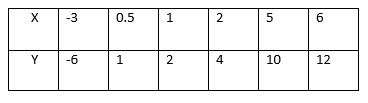This
is the relation which can be written as a set of ordered pairs {(-3,
-6), (0.5, 1), (1, 2), (2, 4), (5, 10), (6, 12)}. The table shows that
the relation satisfies the equation y=2x. The relation R defining the
set of all ordered pairs (x, y) such that y = 2x can be written
symbolically as:
R = {(x, y): y = 2X}.
Relations Between Members in a Set
Find relations between members in a set
Which of the following ordered pairs belong to the relation {(x, y): y>x}?
(1, 2), (2, 1), (-3, 4), (-3, -5), (2, 2), (-8, 0), (-8, -3).
Solution.
(1, 2), (-3, 4), (-8, 0), (8,-3).
Relations Pictorially
Demonstrate relations pictorially
For
example the relation ” is greater than ” involving numbers 1,2,3,4,5
and 6 where 1,3 and 5 belong to set A and 2,4 and 6 belong to set B can
be indicate as follows:-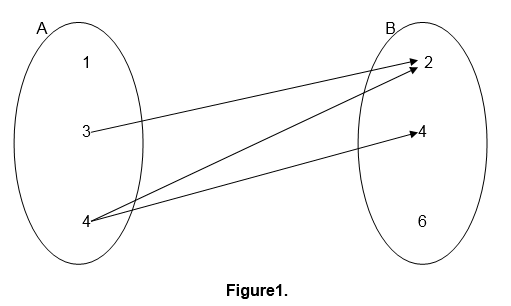This kind of relation representation is referred to as pictorial representation.
Relations
can also be defined in terms of ordered pairs (a,b) for which a is
related to b and a is an element of set A while b is an element of set
B.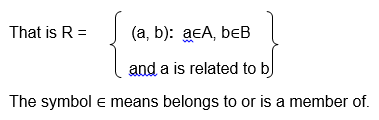For
example the relation ” is a factor of ” for numbers 2,3,5,6,7 and 10
where 2,3,5 and 6 belong to set A and 6,7 and 10 belong to set B can be
illustrated as follows:-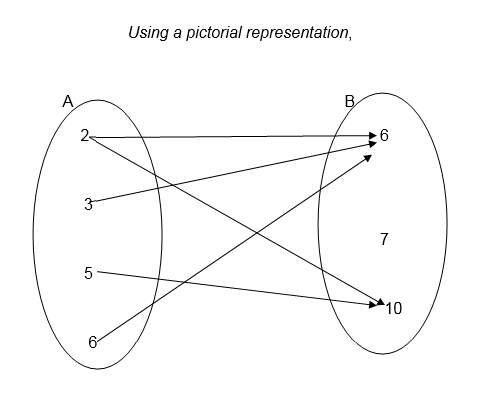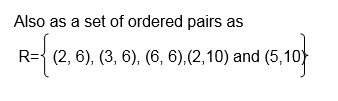Example 1
<!–
[if !supportLists]–>1. Draw an arrow diagram to illustrate the
relation which connects each element of set A with its square.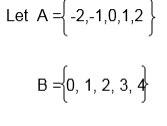Solution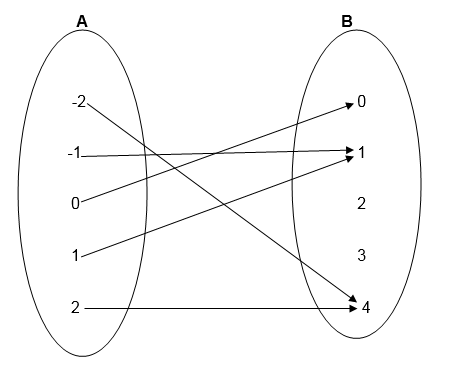Example 2
Using
the information given in example 1, write down the relation in set
notation of ordered pairs. List the elements of ordered pairs.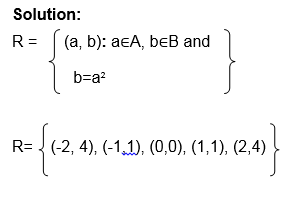Example 3
As we,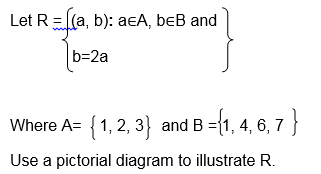Solution;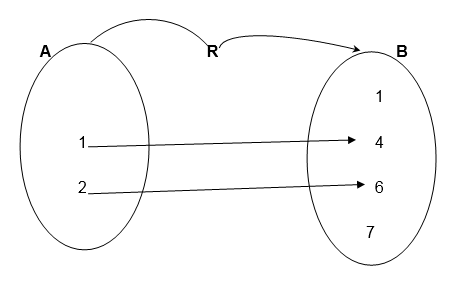Example 4
Let X= {2, 3, 4 } and Y= {3 ,4, 5}
Draw an arrow diagram to illustrate the relation ” is less than”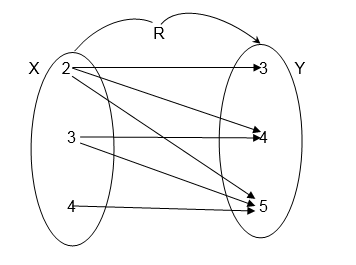Exercise 1
Let P= {Tanzania, China, Burundi, Nigeria}
Draw a pictorial diagram between P and itself to show the relation
“Has a larger population than”
2. Let A = 9,10,14,12 and B = 2,5,7,9 Draw an arrow diagram between A and B to illustrate the relation ” is a multiple of”
3.Let A = mass, Length, time and
B = {Centimeters, Seconds, Hours, Kilograms, Tones}
Use the set notation of ordered pairs to illustrate the relation “Can be measured in”
4.
A group people contain the following; Paul Koko, Alice Juma, Paul
Hassan and Musa Koko. Let F be the set of all first names, and S the set
of all second names.
Draw an arrow diagram to show the connection between F and S
5. Let R={ (x, y): y=x+2}
Where x∈A and A ={ -1,0,1,2}
and y∈B, List all members of set B
Exercise 2
1. Let the relation be defined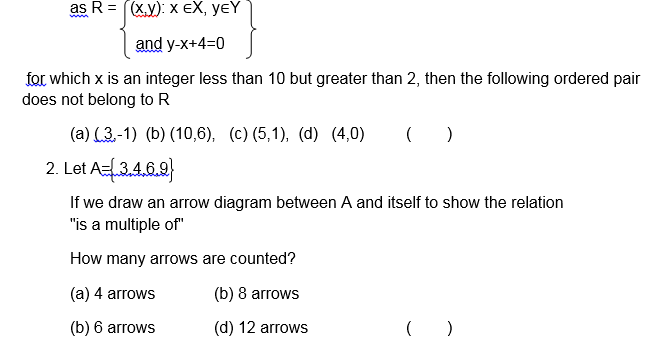Consider the following pictorial diagram representing a relation R.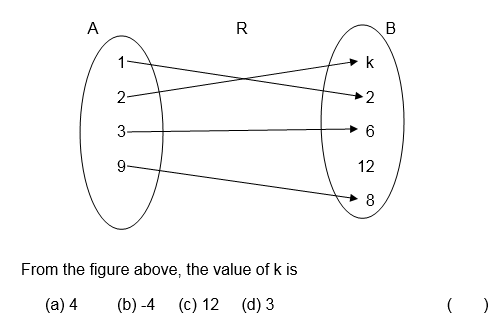Let the relation R be defined asA relation R on sets a and B where A = 1,2,3,4,5 and B = 7,8,9,10,11,12 is defined as ” is a factor of “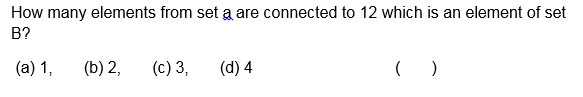1. Hi there to all, how is the whole thing, I think every one is getting more from this website,
and your views are pleasant in favor of new viewers.
0mniartist asmr

2.j.mp

I’m curious to find out what blog platform you are using?
I’m having some minor security problems with my latest website and
I would like to find something more safeguarded.

Do you have any suggestions? asmr 0mniartist

3. Hey! I know this is kinda off topic nevertheless I’d figured
I’d ask. Would you be interested in trading links or maybe guest authoring a blog article or vice-versa?
My site discusses a lot of the same subjects as yours
and I think we could greatly benefit from each other.
If you are interested feel free to shoot me an e-mail.
I look forward to hearing from you! Great
blog by the way! 0mniartist asmr

4. I am no longer sure the place you are getting your information, however
great topic. I must spend a while studying much more or understanding more.
Thanks for excellent info I used to be searching for this
information for my mission. asmr 0mniartist

5. Right away I am ready to do my breakfast, later than having my breakfast coming over again to

6. Unquestionably imagine that that you said. Your favorite justification seemed to be at the web the simplest factor to take into account of.

I say to you, I definitely get annoyed at
the same time as other folks think about issues that they
plainly don’t realize about. You controlled to hit the nail upon the highest as
well as defined out the whole thing without having side-effects , people could take a
signal. Will likely be back to get more. Thanks asmr 0mniartist

7. This site was… how do I say it? Relevant!! Finally I’ve found something that helped me.

Many thanks! asmr 0mniartist

8. Admiring the persistence you put into your site and detailed information you offer.
It’s good to come across a blog every once in a while that isn’t the same old rehashed information. Fantastic read!